Les membres ayant 30 points peuvent parler sur les canaux annonces, projets et hs du chat.
La shoutbox n'est pas chargée par défaut pour des raisons de performances. Cliquez pour charger.

## Forum Casio - Projets de programmation

Index du Forum » Projets de programmation » Mandelbrot Generator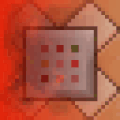Redcmd Hors ligne Membre Points: 341 Défis: 7 Message

#### Mandelbrot Generator

Posté le 15/06/2019 10:38

Hello allô

Default 1x zoom takes 7sec
Max zoom takes around 5-10min
It has a max zoom of 2^50: over one Quadrillion!
Going over 2^48 can be rather buggy
This is because numbers are limited to the 8 byte double variables

Attached file is both SH4 and SH3 compatible: MANDEL.G1A

This does need the 'MonochromeLib' libs the code comes with it now

Controls
[-] Zoom out
[+] Zoom in
[F1] Hide/show HUD which contains Cords, Zoom level and Max Iterations. (Heads Up Display)
[F2] Changes colours of camera rectangle: Black, White & Inverted
[AC] Resets screen back to default state
[EXE] Draw set
[EXIT] Stop drawing the Mandelbrot (If it's taking too long)
[REPLAY] Move camera rectangle around (Arrow Keys: [LEFT], [RIGHT], [UP], [DOWN])

How can I optimize this code to run faster or zoom in further?

#include "fxlib.h"
#include "stdio.h"

#define TRUE 1
#define FALSE 0

typedef enum { ML_TRANSPARENT = -1, ML_WHITE, ML_BLACK, ML_XOR, ML_CHECKER } ML_Color;
typedef char* (*sc_cpv)(void);
const unsigned int sc0135[] = { 0xD201D002, 0x422B0009, 0x80010070, 0x0135 };

unsigned int key;                //pause until key press
int kcode1, kcode2;                //row & col keycode for Bkey_GetKeyWait()
char unused;                    //unused (cause CASIO dumb dumb)
unsigned short dispX, dispY;    //cords on display when drawing mandelbrot

void ML_clear_vram() {
int i, end, * pointer_long, vram;
char* pointer_byte;
end = 4 - vram & 3;
pointer_byte = (char*)vram;
for (i = 0; i < end; i++) pointer_byte[i] = 0;
pointer_long = (int*)(vram + end);
for (i = 0; i < 255; i++) pointer_long[i] = 0;
pointer_byte += 1020 + end;
end = vram & 3;
for (i = 0; i < end; i++) pointer_byte[i] = 0;
}

void ML_display_vram() {
char* LCD_register_selector = (char*)0xB4000000, * LCD_data_register = (char*)0xB4010000, * vram;
int i, j;
for (i = 0; i < 64; i++) {
*LCD_register_selector = 4;
*LCD_data_register = i | 192;
*LCD_register_selector = 4;
*LCD_data_register = 0;
*LCD_register_selector = 7;
for (j = 0; j < 16; j++)
*LCD_data_register = *vram++;
}
}

void ML_display_vram_row(int row) {            //faster than ML_display_vram() which displays the entire screen instead of a single row
unsigned char i;
char* LCD_register_selector = (char*)0xB4000000, *LCD_data_register = (char*)0xB4010000, *vram;
vram = (row << 4) + ML_vram_adress();
*LCD_register_selector = 4;
*LCD_data_register = row | 192;
*LCD_register_selector = 4;
*LCD_data_register = 0;
*LCD_register_selector = 7;
for (i = 0; i < 16; i++)
* LCD_data_register = *vram++;
}

void ML_horizontal_line(int y, int x1, int x2, ML_Color color) {
int i;
char checker;
if (y & ~63 || (x1 < 0 && x2 < 0) || (x1 > 127 && x2 > 127))
return;
if (x1 > x2) {
i = x1;
x1 = x2;
x2 = i;
}
if (x1 < 0)
x1 = 0;
if (x2 > 127)
x2 = 127;
switch (color) {
case ML_BLACK:
if (x1 >> 3 != x2 >> 3) {
vram[(y << 4) + (x1 >> 3)] |= 255 >> (x1 & 7);
vram[(y << 4) + (x2 >> 3)] |= 255 << 7 - (x2 & 7);
for (i = (x1 >> 3) + 1; i < x2 >> 3; i++)
vram[(y << 4) + i] = 255;
} else
vram[(y << 4) + (x1 >> 3)] |= (255 >> (x1 % 8 + 7 - x2 % 8)) << (7 - (x2 & 7));
break;
case ML_WHITE:
if (x1 >> 3 != x2 >> 3) {
vram[(y << 4) + (x1 >> 3)] &= 255 << 8 - (x1 & 7);
vram[(y << 4) + (x2 >> 3)] &= 255 >> 1 + (x2 & 7);
for (i = (x1 >> 3) + 1; i < x2 >> 3; i++)
vram[(y << 4) + i] = 0;
} else
vram[(y << 4) + (x1 >> 3)] &= (255 << 8 - (x1 & 7)) | (255 >> 1 + (x2 & 7));
break;
case ML_XOR:
if (x1 >> 3 != x2 >> 3) {
vram[(y << 4) + (x1 >> 3)] ^= 255 >> (x1 & 7);
vram[(y << 4) + (x2 >> 3)] ^= 255 << 7 - (x2 & 7);
for (i = (x1 >> 3) + 1; i < (x2 >> 3); i++)
vram[(y << 4) + i] ^= 255;
} else
vram[(y << 4) + (x1 >> 3)] ^= (255 >> ((x1 & 7) + 7 - (x2 & 7))) << (7 - (x2 & 7));
break;
case ML_CHECKER:
checker = (y & 1 ? 85 : 170);
if (x1 >> 3 != x2 >> 3) {
vram[(y << 4) + (x1 >> 3)] &= 255 << 8 - (x1 & 7);
vram[(y << 4) + (x2 >> 3)] &= 255 >> 1 + (x2 & 7);
vram[(y << 4) + (x1 >> 3)] |= checker & 255 >> (x1 & 7);
vram[(y << 4) + (x2 >> 3)] |= checker & 255 << 7 - (x2 & 7);
for (i = (x1 >> 3) + 1; i < x2 >> 3; i++)
vram[(y << 4) + i] = checker;
} else {
vram[(y << 4) + (x1 >> 3)] &= (255 << 8 - (x1 & 7)) | (255 >> 1 + (x2 & 7));
vram[(y << 4) + (x1 >> 3)] |= checker & (255 >> (x1 % 8 + 7 - x2 % 8)) << (7 - (x2 & 7));
}
break;
}
}

void ML_vertical_line(int x, int y1, int y2, ML_Color color) {
int i, j;
char checker, byte, * vram = ML_vram_adress();
if (x & ~127 || (y1 < 0 && y2 < 0) || (y1 > 63 && y2 > 63)) return;
if (y1 > y2) {
int tmp = y1;
y1 = y2;
y2 = tmp;
}
if (y1 < 0) y1 = 0;
if (y2 > 63) y2 = 63;

i = (y1 << 4) + (x >> 3);
j = (y2 << 4) + (x >> 3);
switch (color) {
case ML_BLACK:
byte = 128 >> (x & 7);
for (; i <= j; i += 16)
vram[i] |= byte;
break;
case ML_WHITE:
byte = ~(128 >> (x & 7));
for (; i <= j; i += 16)
vram[i] &= byte;
break;
case ML_XOR:
byte = 128 >> (x & 7);
for (; i <= j; i += 16)
vram[i] ^= byte;
break;
case ML_CHECKER:
byte = 128 >> (x & 7);
checker = y1 & 1 ^ x & 1;
for (; i <= j; i += 16) {
if (checker) vram[i] &= ~byte;
else vram[i] |= byte;
checker = !checker;
}
break;
}
}

void ML_pixel(int x, int y, ML_Color color) {
if (x & ~127 || y & ~63) return;
switch (color) {
case ML_BLACK:
vram[(y << 4) + (x >> 3)] |= 128 >> (x & 7);
break;
case ML_WHITE:
vram[(y << 4) + (x >> 3)] &= ~(128 >> (x & 7));
break;
case ML_XOR:
vram[(y << 4) + (x >> 3)] ^= 128 >> (x & 7);
break;
case ML_CHECKER:
if (y & 1 ^ x & 1) vram[(y << 4) + (x >> 3)] &= ~(128 >> (x & 7));
else vram[(y << 4) + (x >> 3)] |= 128 >> (x & 7);
break;
}
}

double divByPow(double n, double x, int p) {        //Divide OR Times n by x, p times (n / x^p): used for numbers bigger than 2^32 (int limit)
if (p < 0)
for (; p < 0; p++)
n *= x;
else
for (; p > 0; p--)
n /= x;
return n;
}

void stop(void) {            //stops drawing set if user presses [EXIT] or [MENU]
if (Bkey_GetKeyWait(&kcode1, &kcode2, 1, 0, 1, &unused))
if (kcode1 == 4 && (kcode2 == 8 || kcode2 == 9)) {
dispX = 128;    //Very hacky stop function
dispY = 64;
}
}

int AddIn_main(int isAppli, unsigned short OptionNum) {        //Main function
unsigned int graphZoom = 1;                //zoom level for graph
char screenZoom;                        //zoom level on screen (rectangle)
int screenX1, screenX2;                    //corner X cords for drawing rectangle to screen
int screenY1, screenY2;                    //corner Y cords for drawing rectangle to screen
unsigned char string;                //Used in converting int/double to char
char HUD = TRUE;                        //Heads Up Display: Cords, Zoom level & Max iteration: toggle with [F1]
char colour = ML_XOR;                    //Colour of rectangle: Black, White or Inverted
int screenX, screenY;                    //offset cords on screen from 0,0 for rectangle
double graphX = 0, graphY = 0;            //cords on graph - where to center mandelbrot
double graphMove;                        //amount graphX & Y changes by when moving rectangle around
int screenMove;                            //amount screenX & Y changes by when moving rectangle around with arrow keys
short tempPixel = 0;                    //Write pixels to temp variable then write the entire 2bytes to VRAM all at once

register double zr, zi;                    //zr is real, zi imaginary
register double zr2, zi2;                //zr2 = zr^2, zi2 = zi^2
register double x1 = -2.0;                //bounding box cords on graph
register double x2 = 2.0;                //bounding box cords on graph
register double y1 = -1.0;                //bounding box cords on graph
register double y2 = 1.0;                //bounding box cords on graph
register double x, y;                    //pixel cords on graph tested if in set
register double xIsz, yIsz;                //amount x/y increases by when ploting graph
register unsigned short iMax = 32;        //max iterations
register unsigned short i;                //iterations

while (TRUE) {

SetTimer(1, 200, stop);
ML_clear_vram();
ML_display_vram();

xIsz = (x2 - x1) / 128;
yIsz = (y2 - y1) / 64;

y = y1;
for (dispY = 0; dispY < 64; dispY++) {
x = x1;
y += yIsz;
for (dispX = 0; dispX < 128; dispX++) {
zr = x;
zi = y;
for (i = 0; i < iMax; i++) {
zr2 = zr * zr;
zi2 = zi * zi;
if (zr2 + zi2 > 4)
break;
zi = zr * zi;
zi += zi + y;
zr = zr2 - zi2 + x;
}
tempPixel = (tempPixel << 1) | (i == iMax);
if ((dispX & 7) == 7)
*vram++ = tempPixel;
x += xIsz;
}
ML_display_vram_row(dispY);
}
SaveDisp(1);
KillTimer(1);
screenX = 0;
screenY = 0;
screenZoom = 1;
Bkey_GetKeyWait(&kcode1, &kcode2, 2, 1, 1, &unused);
do {
GetKey(&key);
screenMove = screenZoom > 4 ? 1 : divByPow(16, 2, screenZoom);
graphMove = screenZoom > 4 ? divByPow(1, 2, graphZoom - (double)screenZoom) : divByPow(16, 2, graphZoom);
switch (key) {
case KEY_CHAR_PLUS:
if (graphZoom < 51) {
graphZoom++;
screenZoom++;
}
break;
case KEY_CHAR_MINUS:
if (graphZoom) {
graphZoom--;
screenZoom--;
}
break;
case KEY_CTRL_UP:
screenY -= screenMove;
graphY -= graphMove;
break;
case KEY_CTRL_DOWN:
screenY += screenMove;
graphY += graphMove;
break;
case KEY_CTRL_LEFT:
screenX -= screenMove;
graphX -= graphMove;
break;
case KEY_CTRL_RIGHT:
screenX += screenMove;
graphX += graphMove;
break;
case KEY_CTRL_F1:
HUD = !HUD;
break;
case KEY_CTRL_F2:
if (colour)
colour--;
else
colour = ML_XOR;
break;
case KEY_CTRL_F3:
//Gray scale, by refreshing screen multiple times per sec at different max iterations (iMax)
break;
case KEY_CTRL_AC:
graphZoom = 1;
graphX = 0;
graphY = 0;
screenZoom = 1;
screenX = 0;
screenY = 0;
key = KEY_CTRL_EXE;
break;
}
RestoreDisp(1);
iMax = 8 * (graphZoom + 3);

if (screenZoom < 8) {
screenX1 = 65 - divByPow(128, 2, screenZoom) + screenX;
screenX2 = 62 + divByPow(128, 2, screenZoom) + screenX;
screenY1 = 32 - (screenZoom > 6 ? 1 : divByPow(64, 2, screenZoom)) + screenY;
screenY2 = 31 + (screenZoom > 6 ? 0 : divByPow(64, 2, screenZoom)) + screenY;
ML_horizontal_line(screenY1, screenX1, screenX2, colour);
ML_horizontal_line(screenY2, screenX1, screenX2, colour);
ML_vertical_line(screenX1 - 1, screenY1, screenY2, colour);
ML_vertical_line(screenX2 + 1, screenY1, screenY2, colour);
} else
ML_pixel(screenX + 64, screenY + 31, colour);

x1 = divByPow(-4, 2, graphZoom) + (0.03125 * graphX);
x2 = divByPow(4, 2, graphZoom) + (0.03125 * graphX);
y1 = divByPow(-2, 2, graphZoom) + (0.03125 * graphY);
y2 = divByPow(2, 2, graphZoom) + (0.03125 * graphY);

if (HUD == TRUE) {
sprintf(&string, "X1:%f", x1);
PrintMini(0, 0, string, 0);
sprintf(&string, "Y1:%f", y1);
PrintMini(0, 6, string, 0);
sprintf(&string, "X2:%f", x2);
PrintMini(81, 53, string, 0);
sprintf(&string, "Y2:%f", y2);
PrintMini(81, 59, string, 0);
sprintf(&string, "MaxI:%u", iMax);
PrintMini(0, 53, string, 0);
if (graphZoom > 32)
sprintf(&string, "Zoom:2^%ux", graphZoom - 1);
else
sprintf(&string, "Zoom:%ux", (int)divByPow(1, 2, -graphZoom + 1));
PrintMini(0, 59, string, 0);
}

ML_display_vram();

} while (key != KEY_CTRL_EXE);
}
return 0;
}

#pragma section _BR_Size
unsigned long BR_Size;
#pragma section
#pragma section _TOP
int InitializeSystem(int isAppli, unsigned short OptionNum) {
}
#pragma section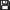Fichier joint

1, 2, 3, 4 ··· 6, 7, 8 SuivanteLephenixnoir En ligne Administrateur Points: 23513 Défis: 149 Message

Citer : Posté le 15/06/2019 15:05 | #

Pretty nice! For reference you might want to try out this other implementation; I don't know how fast it is in practice.

It partially works on my Graph 35+E II, and I really don't know why because the screen isn't compatible. It messes up the display when I leave. Really strange. Not a bug in your code, but I wanted to let you know at least.Not sure why you're using a specific ML_display_vram_row() function since you call it for every row.

I can only suggest one notable optimization right now, which is not using a pixel procedure. You're going to draw the whole screen anyway, so just compute a byte with 8 of these, shifting it every time, then write it to the VRAM in a single instruction when you're done with it. (Larger integers won't work because the VRAM is 1-aligned.)Redcmd Hors ligne Membre Points: 341 Défis: 7 Message

Citer : Posté le 15/06/2019 22:40 | #

Pretty nice! For reference you might want to try out this other implementation; I don't know how fast it is in practice.

Yea I had a look at that, its pretty good for coding it in a single day
It is much faster (like 2x) , but no code download, so IDK why.
His screen stretches over time, I was having the same problem.
Which is why I have a a lot of variables - to keep the number in interger form for as long as possible before changing them into fractions

Not sure why you're using a specific ML_display_vram_row() function since you call it for every row.

I only compute a single row of the set, then push it to the screen - This is so the user can see it as its been drawn, without too much extra delay
ML_display_vram() pushes the entire VRAM to the screen everytime, but only one row is needed
How slow is it to draw to VRAM? compared to saving to temp value and drawing a 8bit value all at once?

(Larger integers won't work because the VRAM is 1-aligned.)

What do you mean it won't work?
VRAM is only contains 2byte variables?
RedCMD#4299 - Discord
Mandelbrot SNKEmini MinesweeperLephenixnoir En ligne Administrateur Points: 23513 Défis: 149 Message

Citer : Posté le 15/06/2019 22:51 | #

I only compute a single row of the set, then push it to the screen - This is so the user can see it as its been drawn, without too much extra delay

Well, makes sense. I couldn't see this unfortunately, probably because of the Graph 35+E II display.

How slow is it to draw to VRAM? compared to saving to temp value and drawing a 8bit value all at once?

Note that VRAM is just normal memory, so access time is the same.

It just happens to be useless to read a byte from the VRAM, perform a shift, set a single bit, write this byte again, only to do the same thing again 7 times with the same byte. If you want a rough estimation of the speed, for pure drawing I'd say my method is 5 to 10 times faster. This is because for each bit, you just have to shift one place then or, then write the whole byte at the end (< 3 cycles per pixel no matter what), whereas you currently execute a complete function call for each pixel, and you even compute the vram offset (> 20 cycles at best). This is also not needed because you traverse the VRAM in memory order.

What do you mean it won't work?

The VRAM is a 1024-byte region of memory. However, you can only perform longword accesses on addresses that are multiples of 4. There are 16 bytes per row, it would be immensely natural that the first byte of the VRAM be located at an address multiple of 4 (or even 16) so that each row spans 4 consecutive longwords.

However, the first byte of the VRAM is located on an address which is 1-aligned, meaning that it's equal to 1 modulus 4. You can't perform a longword access, nor even a word access, at this address. This makes all optimizations using longwords overly sophisticated just because you don't have a disjoint set of longwords for each row. You also can't use large block sizes with the DMA. Honestly, it's completely ridiculous.Redcmd Hors ligne Membre Points: 341 Défis: 7 Message

Citer : Posté le 15/06/2019 23:26 | # |Fichier joint

I updated the code
Didn't seem to make much difference

Drakalex007's mandelbrot is much faster, but I have no idea why
I even tried by changing all doubles to floats and only drawing to the screen after everythings computed
RedCMD#4299 - Discord
Mandelbrot SNKEmini MinesweeperLephenixnoir En ligne Administrateur Points: 23513 Défis: 149 Message

Citer : Posté le 16/06/2019 00:05 | #

(Since markup interpretation is disabled in code blocks, I moved the plain version in your post.)

Obviously most of the time is spent on the sequence computation, not the rendering. For reference though, I meant to optimize it further.

After computing the sequence, you can remove j and just do this:

tempPixel = (tempPixel << 1) | (i == iMax);

Also, when you have filled your byte, you don't have to care about the VRAM offset which you traverse in order. Assuming you set the VRAM pointer at the beginning of the drawing, the function call boils down to:

*vram++ = tempPixel;

You don't even need to reset tempPixel or even call ML_vram_address() which itself performs a syscall.

I'm really unsure why you have this much difference for the same number of iterations. You might want to make these crucial doubles local to use registers instead of placing everything in memory.Redcmd Hors ligne Membre Points: 341 Défis: 7 Message

Citer : Posté le 16/06/2019 03:15 | #

I did all that: much cleaner code nowBut how do I create variables in registers instead of main memory?
RedCMD#4299 - Discord
Mandelbrot SNKEmini MinesweeperLephenixnoir En ligne Administrateur Points: 23513 Défis: 149 Message

Citer : Posté le 16/06/2019 03:45 | #

Redcmd a écrit :
But how do I create variables in registers instead of main memory?

You don't; you just declare variables. Then compiler then chooses where to put them.

The thing is, global variables can only be in memory. By declaring them local you can give the compiler an opportunity to put them into registers, making the code ever slightly more efficient. In old times there was a register keyword for it. It's obsolete and ignored now, but so is the SDK's compiler, so you might as well give it a try.

Now honestly I don't think that's going to make any serious difference, but I can't seem to find anything sub-optimized in your code. Also using local variables is generally cleaner.

Just to be sure, the only thing you are trying to optimize is the critical loop of the drawing here?

SetTimer(1, 200, stop);
ML_clear_vram();
ML_display_vram();
xInz = (x2 - x1) / 128;
yInz = (y2 - y1) / 64;
y = y1;
for (dispY = 0; dispY < 64; dispY++) {
x = x1;
y += yInz;
for (dispX = 0; dispX < 128; dispX++) {
zr = 0;
zi = 0;
zr2 = 0;
zi2 = 0;
for (i = 0; i < iMax; i++) {
zi = zr * zi;
zi += zi + y;
zr = zr2 - zi2 + x;
zr2 = zr * zr;
zi2 = zi * zi;
if (zr2 + zi2 > 4)
break;
}
tempPixel = (tempPixel << 1) | (i == iMax);
if ((dispX & 7) == 7)
* vram++ = tempPixel;
x += xInz;
}
ML_display_vram_row(dispY);
}Redcmd Hors ligne Membre Points: 341 Défis: 7 Message

Citer : Posté le 16/06/2019 04:19 | #

Just to be sure, the only thing you are trying to optimize is the critical loop of the drawing here?

Yup
Nothing else needs optimizing: just the critical loop

I had to declare the doubles in the main function to mark them as register

Oh maybe he only computes half the set and just mirrors it
I could do that, though it woudn't help when its off center
(This doesn't seem to be the case, only showing half the set on screen is still 2x faster)

Ajouté le 16/06/2019 à 22:51 :
Is there any way to 'decompile' a .g1a file?
and if so, how hard would it be to see how it was original coded?
RedCMD#4299 - Discord
Mandelbrot SNKEmini MinesweeperLephenixnoir En ligne Administrateur Points: 23513 Défis: 149 Message

Citer : Posté le 16/06/2019 22:55 | #

It is possible to get to the assembler source, but reconstructing the original algorithm would be rather hard. If FPU operations are inlined, that'd be hellish; otherwise I'd estimate that as several hours to understand the structure and probably a few more for the details. If you haven't used SuperH assembler before then you need to get accustomed to that as well. All in all it's pretty impractical.Redcmd Hors ligne Membre Points: 341 Défis: 7 Message

Citer : Posté le 18/06/2019 01:42 | #

Do you know if squaring a number is faster than general multiplication?
(x * x) vs (x * y)?
like if it detects if both numbers are the same, it runs a slightly faster circuit/function?
cause I have an idea to replace one of the mults with a squarer, but I think it adds another subtraction, which unless squaring is faster, the sub will slow it down
RedCMD#4299 - Discord
Mandelbrot SNKEmini MinesweeperLephenixnoir En ligne Administrateur Points: 23513 Défis: 149 Message

Citer : Posté le 18/06/2019 02:11 | #

Unfortunately no, there's no squaring instruction in the processor. Multiplication is the same regardless of the operands.Redcmd Hors ligne Membre Points: 341 Défis: 7 Message

Citer : Posté le 26/06/2019 03:31 | #

Would using Gint make it faster?
is it better at optimizations or is it draw functions faster than monoChromeLib?
RedCMD#4299 - Discord
Mandelbrot SNKEmini MinesweeperLephenixnoir En ligne Administrateur Points: 23513 Défis: 149 Message

Citer : Posté le 26/06/2019 03:49 | #

gint's drawing functions are ultimately faster than MonochromeLib, however using your own access to the VRAM with the bit shifting strategy from earlier is the definite fastest way I can imagine.

Just an intuition: the range of the sequence should be pretty predictable and restricted when you're viewing the whole fractal. Could it be possible to use fixed-point arithmetic there?Redcmd Hors ligne Membre Points: 341 Défis: 7 Message

Citer : Posté le 26/06/2019 23:26 | #

How could I make it fixed point?
can the fixed point be change after being declared?
I'm still confused about how it's able to zoom in 2^48 times
because 32bit floating point numbers only have 27bits of precision yet it seems to be able to hold 2.0 and 0.00000000001 (2^-48) at the same time (2.0000000000000000001 (2+2^-48) (16ish 0's))
some number 'should' be able to be fixed point
x, x1, y, y1 could be fixed so the max number is between 4 and 8
yInz, xInz is never bigger than 2^-4 but 'could' be as small as 2^-48 (xInz => Increase 'x' by xInz 128 times)
RedCMD#4299 - Discord
Mandelbrot SNKEmini MinesweeperLephenixnoir En ligne Administrateur Points: 23513 Défis: 149 Message

Citer : Posté le 26/06/2019 23:35 | #

You could use 64-bit fixed point, maybe something like 8:56 or 4:60. This range is large enough for a decent exploration of the fractal, and you can make it much faster than floating-point because it doesn't have to be implemented in software.Redcmd Hors ligne Membre Points: 341 Défis: 7 Message

Citer : Posté le 27/06/2019 00:00 | #

64bit?
what variable handles 64bits?
cause in my testing I found; int, long int and floats to be only be 16bits
and doubles and long doubles 32bits
RedCMD#4299 - Discord
Mandelbrot SNKEmini MinesweeperLephenixnoir En ligne Administrateur Points: 23513 Défis: 149 Message

Citer : Posté le 27/06/2019 00:10 | #

You have long long int or better, int64_t, which are 64-bit variables. GCC knows them well, I think the SDK might as well. Worst case you can use a bit of assembler.Redcmd Hors ligne Membre Points: 341 Défis: 7 Message

Citer : Posté le 27/06/2019 01:07 | #

The SDK doesn't surport any of them
D:\Documents\CASIO\fx-9860G SDK\MandelBrot\Mandel.c(6) : C2102 (E) Illegal type combination
D:\Documents\CASIO\fx-9860G SDK\MandelBrot\Mandel.c(7) : C2500 (E) Illegal token "tests"
RedCMD#4299 - Discord
Mandelbrot SNKEmini MinesweeperLephenixnoir En ligne Administrateur Points: 23513 Défis: 149 Message

Citer : Posté le 27/06/2019 01:19 | #

Rooh, that's C89 for you. Sorta depressing. o(>_>)o

I'm more and more puzzled by what Drakalex has done. He's a skilled programmer but he's not into grinding optimizations. Did we miss something obvious?Redcmd Hors ligne Membre Points: 341 Défis: 7 Message

Citer : Posté le 27/06/2019 01:26 | #

Maybe he's only using floats, not doubles
Only calcuating one half of the set and just flipping the image (because top and bottom of the set is the same)
tho, even when rendering just the top half of the set his is still faster
maybe he is using fixed point?
he is only displaying the pic after the set is calculated, but I tried that too, but that didn't change much
by default the mex iterations is set at 50 on his and 32 on my
RedCMD#4299 - Discord
Mandelbrot SNKEmini Minesweeper
1, 2, 3, 4 ··· 6, 7, 8 Suivante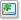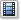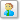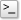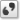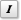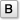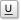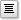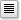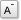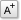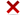Alignement de l'image: Redimensionnement de l'image (en pixel):
Afficher la liste des membres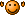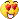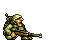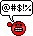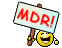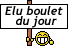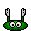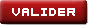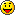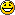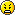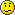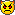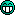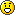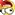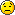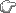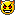Σ π θ ± α β γ δ Δ σ λ
Veuillez donner la réponse en chiffre
Vous devez activer le Javascript dans votre navigateur pour pouvoir valider ce formulaire.

Si vous n'avez pas volontairement désactivé cette fonctionnalité de votre navigateur, il s'agit probablement d'un bug : contactez l'équipe de Planète Casio.

Planète Casio v4.3 © créé par Neuronix et Muelsaco 2004 - 2023 | Il y a 64 connectés | Nous contacter | Qui sommes-nous ? | Licences et remerciements

Planète Casio est un site communautaire non affilié à Casio. Toute reproduction de Planète Casio, même partielle, est interdite.
Les programmes et autres publications présentes sur Planète Casio restent la propriété de leurs auteurs et peuvent être soumis à des licences ou copyrights.
CASIO est une marque déposée par CASIO Computer Co., Ltd